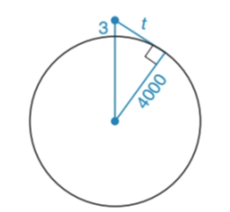Chapter 6.2, Problem 27E### Elementary Geometry for College St...

6th Edition
Daniel C. Alexander + 1 other
ISBN: 9781285195698

#### Solutions

Chapter
Section### Elementary Geometry for College St...

6th Edition
Daniel C. Alexander + 1 other
ISBN: 9781285195698
Textbook Problem
1 views

# An airplane reaches an altitude of 3 mi above the earth. Assuming a clear day and that a passenger has binoculars, how far can the passengers see?(HINT: The radius of the earth is approximately 4000 mi.)To determine

To find:

The distance that the passengers able to see.

Explanation

Given:

Calculation:

The place reaches an altitude 3mi above the earth.

In the given figure we can observe the right triangle.

By Pythagorean theorem.

(4000+3)2=40002+t2(4003)2=4000</

### Still sussing out bartleby?

Check out a sample textbook solution.

See a sample solution

#### The Solution to Your Study Problems

Bartleby provides explanations to thousands of textbook problems written by our experts, many with advanced degrees!

Get Started

#### Prove, using Definition 9, that limxx3=. Definition 9

Single Variable Calculus: Early Transcendentals, Volume I

#### In Exercises 69-74, rationalize the numerator. 69. 2x3

Applied Calculus for the Managerial, Life, and Social Sciences: A Brief Approach

#### In Problems 1-6, find the absolute maxima and minima for on the interval .

Mathematical Applications for the Management, Life, and Social Sciences

#### True or False: converges mean exists.

Study Guide for Stewart's Multivariable Calculus, 8th

#### What is the partial fraction form of ?

Study Guide for Stewart's Single Variable Calculus: Early Transcendentals, 8th